Capacitor Guide

# What are impedance/ ESR frequency characteristics in capacitors?

The basics of capacitors are explained in this technical column.
Today's column describes frequency characteristics of the amount of impedance |Z| and equivalent series resistance (ESR) in capacitors.

Understanding frequency characteristics of capacitors enables you to determine, for example, the noise suppression capabilities or the voltage fluctuation control capabilities of a power supply line. Frequency characteristics are therefore important parameters that are essential for circuit design. This column describes two types of frequency characteristics: impedance |Z| and ESR.

## 1. Frequency characteristics of capacitors

The impedance Z of an ideal capacitor (Fig. 1) is shown by formula (1), where ω is the angular frequency and C is the electrostatic capacitance of the capacitor.

From formula (1), the amount of impedance |Z| decreases inversely with the frequency, as shown in Figure 2. In an ideal capacitor, there is no loss and the equivalent series resistance (ESR) is zero.

In actual capacitors (Fig. 3), however, there is some resistance (ESR) from loss due to dielectric substances, electrodes or other components in addition to the capacity component C and some parasitic inductance (ESL) due to electrodes, leads and other components. As a result, the frequency characteristics of |Z| form a V-shaped curve (or U-shaped curve depending on the type of capacitor) as shown in Figure 4, and ESR also shows frequency characteristics for values equivalent to loss.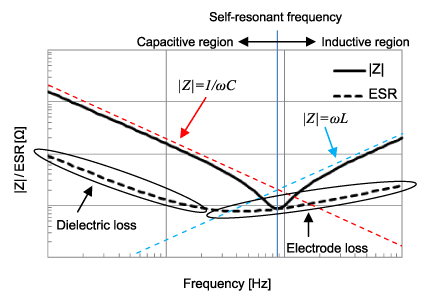Figure 4. An example of |Z|/ESR frequency characteristics of an actual capacitor

The reason why |Z| and ESR form curves like those shown in Figure 4 can be explained as follows.

### Low-frequency region:

|Z| in regions with a low frequency decreases inversely with frequency, similar to the ideal capacitor. ESR shows a value equivalent to dielectric loss from delay of polarization in the dielectric substance.

### Near the resonance point:

As the frequency rises, ESR resulting from parasitic inductance, electrode resistivity and other factors causes |Z| behavior to stray from that of an ideal capacitor (red broken line) and reach a minimum value. The frequency at which |Z| is the minimum value is called the self-resonant frequency, and at this time, |Z|=ESR. Once the self-resonant frequency is exceeded, the element characteristic changes from capacitor to inductor, and |Z| starts to increase. The region below the self-resonant frequency is called the capacitive region and the region above is called the inductive region.

ESR is affected by loss caused by the electrode in addition to dielectric loss.

### High-frequency region:

In frequency zones even higher than the resonance point, |Z| characteristics are determined by parasitic inductance (L). |Z| in the high-frequency region approaches formula (2) and increases proportionately with frequency.

As for ESR, electrode skin effects, proximity effects and other effects begin to appear.

The above was an explanation of frequency characteristics of an actual capacitor. The main point to remember is that, as frequency rises, ESR and ESL cannot be ignored. As there is an increasing number of applications in which capacitors are used at high frequencies, ESR and ESL become an important parameter that shows capacitor performance, in addition to electrostatic capacitance values.

## 2. Frequency characteristics of different types of capacitors

The previous section explained that parasitic components of capacitors such as ESR and ESL greatly affect their frequency characteristics. As the types of parasitic components vary with the type of capacitor, let's look at the different frequency characteristics of different types of capacitors.

Figure 5 shows the |Z| and ESR frequency characteristics of various capacitors with an electrostatic capacitance of 10 uF. Except for the film capacitor, all the capacitors are SMD types.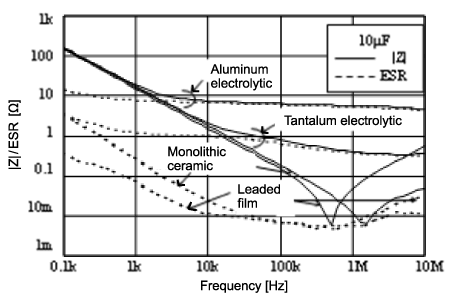Figure 5. |Z|/ ESR frequency characteristics of different types of capacitors

As the electrostatic capacitance is 10 uF in all the capacitors shown in Figure 5, the |Z| value is the same for all types in the capacitive region at frequencies under 1 kHz. Rising above 1 kHz, |Z| values increase much higher in the aluminum electrolytic capacitor and the tantalum electrolytic capacitor than in the multilayer ceramic capacitor and the film capacitor. This is because there is high resistivity of the electrolyte material and large ESR in the aluminum electrolytic capacitor and tantalum electrolytic capacitor. The film capacitor and multilayer ceramic capacitor use metallic materials for their electrodes, and therefore exhibit very minimal ESR.

The multilayer ceramic capacitor and leaded film capacitor show roughly the same characteristics up to the resonance point, but the self-resonant frequency is higher and |Z| in the inductive region is lower in the multilayer ceramic capacitor. This is because, in leaded film capacitors, the inductance is only as large as that due to the lead wire.

These results show that impedance is small over a wide frequency band in SMD-type multilayer ceramic capacitors, making them the best-suited capacitors for high-frequency applications.

## 3. Frequency characteristics of multilayer ceramic capacitors

There are also different types of multilayer ceramic capacitors made of different materials and with different shapes. Let's look at how these factors affect frequency characteristics.

(1) ESR
ESR in the capacitive region depends on dielectric loss caused by the dielectric material. Class 2 high dielectric constant materials tend to have higher ESR levels as they use ferroelectrics. Class 1 temperature compensation materials use paraelectrics, and therefore exhibit very little dielectric loss and have low ESR levels.
In addition to the resistivity of the electrode material, the shape of the electrode (thickness, length, width) and the number of layers, ESR levels at high frequencies from around the resonance point to the inductive region are also influenced by the skin effect and by the proximity effect. Ni is often used as the electrode material, but Cu that has low resistivity is sometimes chosen for low loss-type capacitors.

(2) ESL
ESL of multilayer ceramic capacitors is strongly affected by the internal electrode structure. Where the size of the internal electrode is shown as length l, width w and thickness d, the inductance ESL of the electrode can be shown by formula (3), according to F. W. Grover.

From this formula, it is apparent that ESL decreases as capacitor electrodes become shorter, wider and thicker.

Figure 6 shows the relationship between the nominal capacity and self-resonant frequency for different sizes of multilayer ceramic capacitors. You can see that, as size decreases, self-resonant frequency increases and ESL decreases for the same capacity. This means that small capacitors with a short length l are best-suited to high-frequency applications.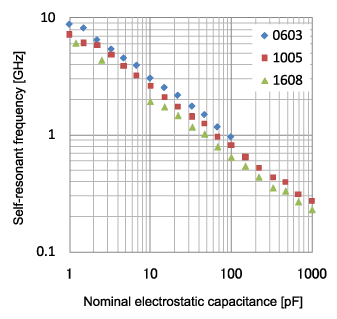Figure 6. The relationship between nominal capacity and self-resonant frequency for different sizes

Figure 7 shows an LW reverse capacitor with a short length l and large width w. From the frequency characteristics shown in Figure 8, you can see that LW reverse capacitors have lower impedance and better characteristics than a conventional capacitor of the same capacity. By using LW reverse capacitors, the same performance can be achieved as that of conventional capacitors with a fewer number of units. The reduction of unit number enables reduced costs and a reduction of mounting space.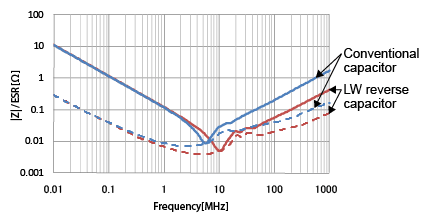Figure 8. |Z|/ESR of an LW reverse capacitor and a general-purpose capacitor

## 4. How to obtain frequency characteristics data

While data on frequency characteristics can be obtained using an impedance analyzer or vector network analyzer, such data is also now available on the websites of parts manufacturers.

Figure 9 shows a screen view of Murata's "SimSurfing" design tool. Characteristics can be displayed by simply entering the model number and the items you wish to check. Furthermore, you can download SPICE network lists and S2P data as data for simulations. Feel free to use these for design of all types of electronic circuits.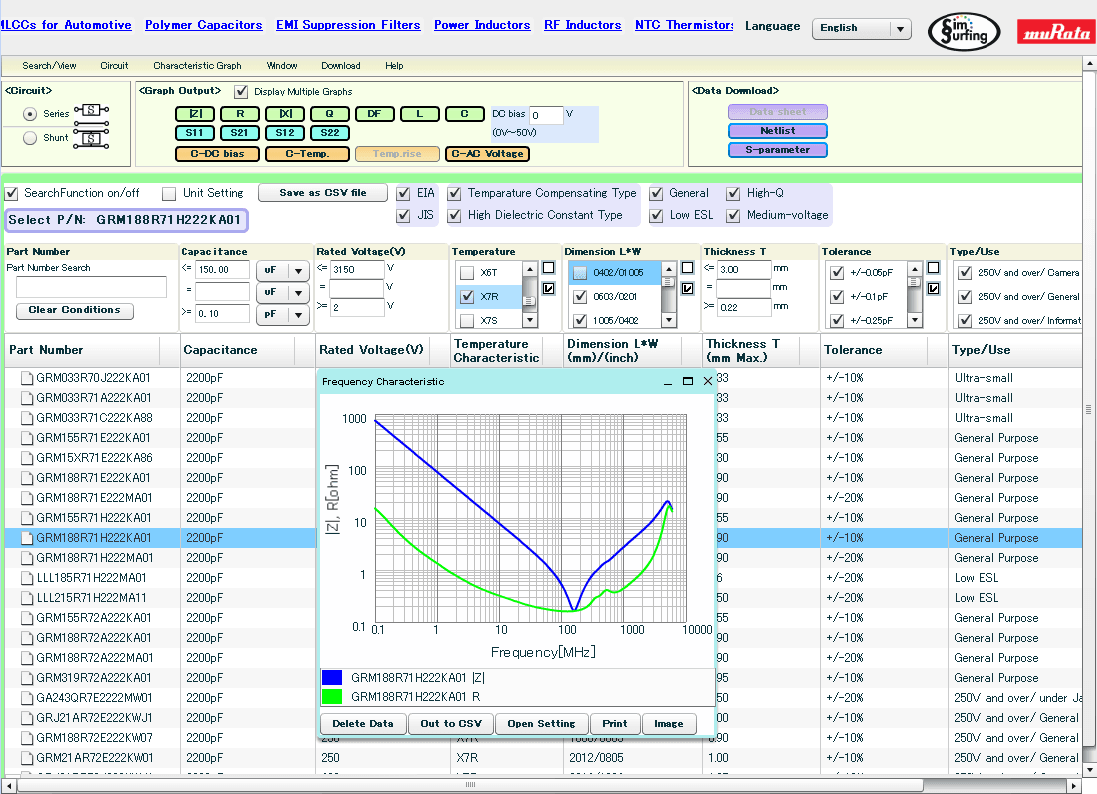Figure 9. An example screen view of the "SimSurfing" design tool （Click the image to see the enlarged image）

See here for SimSurfing

Person in charge: A.S., Components Business Unit, Murata Manufacturing Co., Ltd.